The Australian Journal of Mathematical Analysis and Applications

 Home News Editors Volumes RGMIA Subscriptions Authors Contact

ISSN 1449-5910

You searched for bello
Total of 15 results found in site

4: Paper Source PDF document

Paper's Title:

Solving Two Point Boundary Value Problems by Modified Sumudu Transform Homotopy Perturbation Method

Author(s):

Asem AL Nemrat and Zarita Zainuddin

School of Mathematical Sciences,
Universiti Sains Malaysia,
11800 Penang,
Malaysia.
E-mail: alnemrata@yahoo.com
zarita@usm.my

Abstract:

This paper considers a combined form of the Sumudu transform with the modified homotopy perturbation method (MHPM) to find approximate and analytical solutions for nonlinear two point boundary value problems. This method is called the modified Sumudu transform homotopy perturbation method (MSTHPM). The suggested technique avoids the round-off errors and finds the solution without any restrictive assumptions or discretization. We will introduce an appropriate initial approximation and furthermore, the residual error will be canceled in some points of the interval (RECP). Only a first order approximation of MSTHPM will be required, as compared to STHPM, which needs more iterations for the same cases of study. After comparing figures between approximate, MSTHPM, STHPM and numerical solutions, it is found through the solutions we have obtained that they are highly accurate, indicating that the MSTHPM is very effective, simple and can be used to solve other types of nonlinear boundary value problems (BVPs).

3: Paper Source PDF document

Paper's Title:

MSplit Equality for Monotone Inclusion Problem and Fixed Point Problem in Real Banach Spaces

Author(s):

1,2Christian Chibueze Okeke, 3Abdumalik Usman Bello, 1Chinedu Izuchukwu, and 1Oluwatosin Temitope Mewomo

1School of Mathematics,
Statistics and Computer Science,
University of KwaZulu-Natal, Durban,
South Africa.
E-mail: okekec@ukzn.ac.za
E-mail: izuchukwuc@ukzn.ac.za
E-mail: mewomoo@ukzn.ac.za

2DST-NRF Center of Excellence in Mathematical and Statistical Sciences (CoE-Mass)
Johannesburg,
South Africa.

3Federal University,
Dutsin-Ma, Katsina State,
Nigeria.
E-mail: uabdulmalik@fudutsinma.edu.ng

Abstract:

In this paper a new iterative algorithm for approximating a common solution of split equality monotone inclusion problem and split equality fixed point problem is introduced. Using our algorithm, we state and prove a strong convergence theorem for approximating an element in the intersection of the set of solutions of a split equality monotone inclusion problem and the set of solutions of a split equality fixed point problem for right Bregman strongly nonexpansive mappings in the setting of p-uniformly convex Banach spaces which are also uniformly smooth. We also give some applications.

2: Paper Source PDF document

Paper's Title:

A New Hardy-Hilbert's Type Inequality for Double Series and its Applications

Author(s):

Mingzhe Gao

Department of Mathematics and Computer Science, Normal College Jishou University,
Jishou Hunan, 416000,
People's Republic of China
mingzhegao1940@yahoo.com.cn

Abstract:

In this paper, it is shown that a new Hardy-Hilbert’s type inequality for double series can be established by introducing a parameter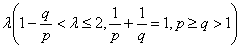and the weight function of the form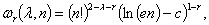where c is Euler constant and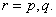And the coefficient is proved to be the best possible. And as the mathematics aesthetics, several important constants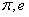and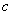appear simultaneously in the coefficient and the weight function when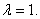In particular, for case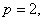some new Hilbert’s type inequalities are obtained. As applications, some extensions of Hardy-Littlewood’s inequality are given.

1: Paper Source PDF document

Paper's Title:

On Pseudo Almost Periodic Solutions to Some Neutral Functional-Differential Equations

Author(s):

Toka Diagana and Eduardo Hernández

Department of Mathematics, Howard University
2441 6th Street NW, Washington DC 20059,
USA.
tdiagana@howard.edu

Departamento de Matemática, I.C.M.C. Universidade de Săo Paulo,
Caixa Postal 668, 13560-970, Săo Carlos SP,
Brazil.
lalohm@icmc.sc.usp.br

Abstract:

This paper discusses the existence and uniqueness of pseudo almost periodic solutions to a class of partial neutral functional-differential equations. Under some suitable assumptions, existence and uniqueness results are obtained. An example is given to illustrate abstract results.

1: Paper Source PDF document

Paper's Title:

A Dynamic Contact Problem for an Electro Viscoelastic Body

Author(s):

Denche M. and Ait Kaki L.

Laboratoire Equations Differentielles,
Departement de Mathematiques,
Universite Constantine 1,
Algeria.

Ecole Normale Superieure,
Departement des Sciences Exactes et Informatique,
Plateau Mansourah, Constantine.
Algeria.

Abstract:

We consider a dynamic problem which describes a contact between a piezoelectric body and a conductive foundation. The frictionless contact is modelled with the normal compliance, the electric conditions are supposed almost perfect. We prove the existence of a unique weak solution for almost perfect electric contact.

1: Paper Source PDF document

Paper's Title:

Strong Convergence Theorem for a Common Fixed Point of an Infinite Family of J-nonexpansive Maps with Applications

Author(s):

Charlse Ejike Chidume, Otubo Emmanuel Ezzaka and Chinedu Godwin Ezea

African University of Science and Technology,
Abuja,
Nigeria.
E-mail: cchidume@aust.edu.ng

Ebonyi State University,
Abakaliki,
Nigeria.
E-mail: mrzzaka@yahoo.com

Nnamdi Azikiwe University,
Awka,
Nigeria.
E-mail: chinedu.ezea@gmail.com

Abstract:

Let E be a uniformly convex and uniformly smooth real Banach space with dual space E*. Let {Ti}i=1 be a family of J-nonexpansive maps, where, for each i,~Ti maps E to 2E*. A new class of maps, J-nonexpansive maps from E to E*, an analogue of nonexpansive self maps of E, is introduced. Assuming that the set of common J-fixed points of {Ti}i=1 is nonempty, an iterative scheme is constructed and proved to converge strongly to a point x* in n=1FJTi. This result is then applied, in the case that E is a real Hilbert space to obtain a strong convergence theorem for approximation of a common fixed point for an infinite family of nonexpansive maps, assuming existences. The theorem obtained is compared with some important results in the literature. Finally, the technique of proof is also of independent interest.

1: Paper Source PDF document

Paper's Title:

Strong Convergence Theorems for a Common Zero of an Infinite Family of Gamma-Inverse Strongly Monotone Maps with Applications

Author(s):

Charles Ejike Chidume, Ogonnaya Michael Romanus, and Ukamaka Victoria Nnyaba

African University of Science and Technology, Abuja,
Nigeria.
E-mail: cchidume@aust.edu.ng
E-mail: romanusogonnaya@gmail.com
E-mail: nnyabavictoriau@gmail.com

Abstract:

Let E be a uniformly convex and uniformly smooth real Banach space with dual space E* and let Ak:EE*, k=1, 2, 3 , ...
be a family of inverse strongly monotone maps such that k=1 Ak-1(0)≠∅.
A new iterative algorithm is constructed and proved to converge strongly to a common zero of the family.
As a consequence of this result, a strong convergence theorem for approximating a common J-fixed point for an infinite family of
gamma-strictly J-pseudocontractive maps is proved. These results are new and improve recent results obtained for these classes of nonlinear maps.
Furthermore, the technique of proof is of independent interest.

1: Paper Source PDF document

Paper's Title:

Iterative Algorithm for Split Generalized Mixed Equilibrium Problem Involving Relaxed Monotone Mappings in Real Hilbert Spaces

Author(s):

1U.A. Osisiogu, F.L. Adum, and 2C. Izuchukwu

1Department of Mathematics and Computer Science,
Ebonyi State University, Abakaliki,
Nigeria.

2School of Mathematics, Statistics and Computer Science,
University of KwaZulu-Natal, Durban,
South Africa.
E-mail: izuchukwuc@ukzn.ac.za, izuchukwu_c@yahoo.com

Abstract:

The main purpose of this paper is to introduce a certain class of split generalized mixed equilibrium problem involving relaxed monotone mappings. To solve our proposed problem, we introduce an iterative algorithm and obtain its strong convergence to a solution of the split generalized mixed equilibrium problems in Hilbert spaces. As special cases of the proposed problem, we studied the proximal split feasibility problem and variational inclusion problem.

1: Paper Source PDF document

Paper's Title:

Some Convergence Results for  Jungck-Am Iterative Process In Hyperbolic Spaces

Author(s):

Akindele Adebayo Mebawondu and Oluwatosin Temitope Mewomo

School of Mathematics, Statistics and Computer Science,
University of KwaZulu-Natal, Durban,
South Africa.
E-mail: 216028272@stu.ukzn.ac.za, mewomoo@ukzn.ac.za

Abstract:

In this paper, we introduce a new three steps iterative process called Jungck-AM iterative process and show that the proposed iterative process can be used to approximate fixed points of Jungck-contractive type mappings and Jungck-Suzuki type mappings. In addition, we establish some strong and Δ-convergence results for the approximation of fixed points of Jungck-Suzuki type mappings in the frame work of uniformly convex hyperbolic space. Furthermore, we show that the newly proposed iterative process has a better rate of convergence compare to the Jungck-Noor, Jungck-SP, Jungck-CR and some existing iterative processes in the literature. Finally, stability, data dependency results for Jungck-AM iterative process is established and we present an analytical proof and numerical examples to validate our claim.

Search and serve lasted 1 second(s).